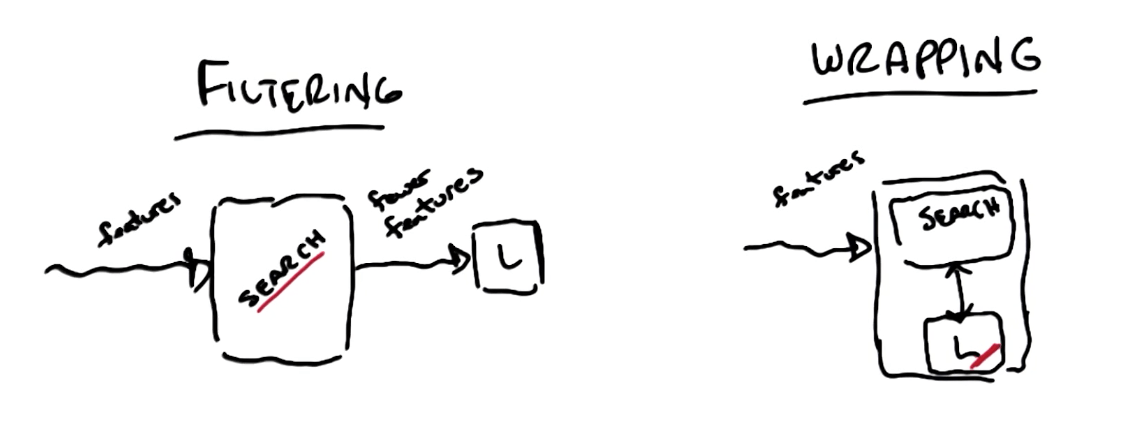Feature engineering and scaling with scikit-learn.
Feature Engineering and Scaling

## Feature Engineering: Scaling and Selection¶

Feature Scaling

• Formula
• $$X' = \frac {X - X_{min}}{X_{max} - X_{min}}$$

Algorithms affected by feature rescaling

• Algorithms in which two dimensions affect the outcome will be affected by rescaling
• SVM with RBF kernel
• When you maximize the distance, you've 2 or more dimensions
• Think of the x and y axis with different dimensions and you need to calculate the distance
• K-means clustering

Feature Scaling Manually in Python

In :
### FYI, the most straightforward implementation might
### throw a divide-by-zero error, if the min and max values are the same
### data point has the same value for that feature!
### why would you rescale it?  Or even use it at all?
def featureScaling(arr):

max_num = max(arr)
min_num = min(arr)

lst = []

for num in arr:
X_prime = (num - min_num) / (max_num - min_num)
lst.append(X_prime)

return lst

# tests of your feature scaler--line below is input data
data = [115, 140, 175]
print(featureScaling(data))

[0.0, 0.4166666666666667, 1.0]


Feature Scaling in Scikit-learn

In :
from sklearn.preprocessing import MinMaxScaler
import numpy as np

In :
# 3 different training points for 1 feature
weights = np.array([, , ]).astype(float)

In :
# Instantiate
scaler = MinMaxScaler()

In :
# Rescale
rescaled_weights = scaler.fit_transform(weights)
rescaled_weights

Out:
array([[ 0.        ],
[ 0.41666667],
[ 1.        ]])

Feature Selection

• Why do we want to select features?
• Knowledge discovery
• Interpretability
• Insight
• Curse of dimensionality

Feature Selection: Algorithms

• How hard is the problem?
• Exponential
• $${n \choose m}$$
• $$2^n$$
• n choose m
• Assuming our original number of features: n
• New number of features: m
• Where m <= n

Filtering vs Wrapping

• No feedback
• Learning algorithm cannot inform on the impact of the changes in features
• Criteria built in search with no reference to the learner
• Ignores learning problem
• You'll look at features in isolation
• Fast
• Slow
• Feedback
• Criteria built in the learner
• Takes into account model bias and learningFiltering: Search Part

• We can use a Decision Tree algorithm for the search function then feed to the learner which does not do well with filtering features
• This is because DTs are good at filtering the best features
• If you want to know all the features, you can easily overfit
• Other generic ways
• Information gain
• Entropy
• "Useful" features
• Independent, non-redundant

Wrapping: Search Part (have to deal with the exponential problem)

• Hill climbing
• Randomized optimization
• Forward
• You pass the features individually to the learning algorithms and get their scores
• You pick
• 2 features
• Choose highest score
• 3 features
• Choose highest score
• If lower than 2 features, stop

Relevance: Measures Effect on BOC

• X_i, feature, is strongly relevant if removing it degrades Bayes Optimal Classifier (BOC)
• BOC is the best you can do on average if you can find it
• X_i, feature, is weakly relevant if
• Not strongly relevant
• Subset of features S such that adding x_i to S improves BOC
• X_i is otherwise irrelevant

Usefulness: Measures Effect on Particular Predictor

• Minimizing error given a model/learner
Tags: